# 2021年成人高考高起点《理数》模拟试题四

2021-03-04 15:07 江西成考网

1.A.{2，5}
B.{3，2}
C.{(2，5)}
D.{(5，2)}

2.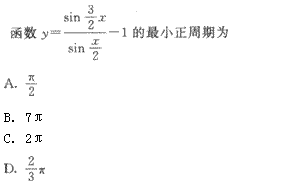A.A
B.B
C.C
D.D

3.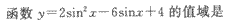（   ）

A.[0，12]
B.[0，11]
C.[-1，1]
D.[5，10]

4.设m<0，那么实数m的三角形式是（　　）

A.B.C.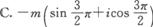D.5.A.M ∪ N={x|x2>4}
B.M ∩ N={x|2<x<3}
C.M ∩ N={x|x<-2}
D.M ∪ N=R

6.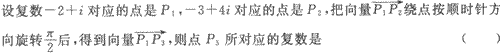A.3+2i
B.3+i
C.1+2i
D.1+3i

7.实数a，b，命题甲：|a+b|=|b|+|b|；命题乙：ab≥0，则有（　　）

A.甲是乙的充分但不必要条件
B.甲是乙的必要但不充分条件
C.甲是乙的充要条件
D.甲是乙的既不充分也不必要条件

8.（   ）

A.充分不必要条件
B.必要不充分条件
C.充要条件
D.既非充分又非必要条件

9.两根之和为6，两根之差的绝对值为8的一元二次方程是（   ）

A.x2-6x+7=0
B.x2+6x+8=0
C.x2+6x-8=0
D.x2-6x-7=0

10.二次曲线x2-y2+28x-14y+47=0的离心率等于（   ）

A.1
B.2
C.√2
D.√2 /2

11.{a}=26,b=(-5,12),且a与b同方向，则a=（   ）

A.（10，24）
B.（10，-24）
C.（-10，24）
D.（5，12）

12.A.B.C.D.13.A.B.C.D.14.已知ab>0,则"x=√ab"是"a,x,b成等比数列"的（   ）

A.充分不必要条件
B.必要不充分条件
C.充要条件
D.既非充分又非必要条件

15.抛物线y=2px2的准线方程是（   ）

A.x=-p/2
B.y=-p/2
C.x=-1/8p
D.y=-1/8p

16.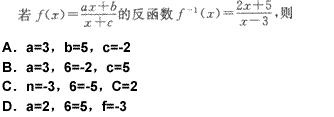A.A
B.B
C.C
D.D

17.已知圆的方程为(x+1)2+(y-4)2=9，过P(2，0)作该圆的一条切线，切点为A，则PA的长度为 （　　）

A.4
B.5
C.10
D.12

18.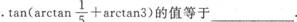19.20.已知i，j，k是彼此互相垂直的单位向量，向量a=3i-2j+k，b=4i+3j-6k，则a·b=__________

21.已知离散型随机变量ζ的分布列为

 ζ 0 1 2 3 4 P 0.3 0.2 0.2 0.1 0.1

22.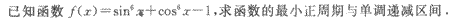23.设两个方程x2-ax+1=0与x2-bx+1=0的四个根组成以2为公比的等比数列，求盘与b的积。

24.25.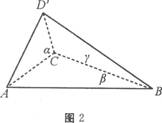437
2021年成人高考考试时间
10月23,24日+